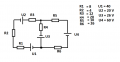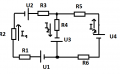# Solving shematics with multiple voltage sources using kirch laws

#### Human2

Joined Dec 22, 2017
51
Okay everyone so this assigment:So okay first thing that I need to do is find the direction of a current in each branch.

I thought that there is a rule for that, and that rule is "(while doing an assigment) current goes from + of voltage source to - of voltage source.

Following that rule, I did this:And from that I wrote these 3 equations:

I2 = I1 + I 3
U1 + U2 + U3 = I1*(R1 + R2 + R3) + I2*R4
U4 + U3 = I3*(R5 + R6) + I2*R4
------------------------------------------------------
I2 = I1 + I 3
86 = 24*I1 + 20*I2
86 = 36*I3 + 20*I2

Did I do everything right here ? Because I got that I2 = -1.654 A
Did I do something wrong with equations or is my math just bad ?

#### Human2

Joined Dec 22, 2017
51
Oh so my math is wrong then... On my way home I first calculated that I2 is -1.6 A, calculated again and got then 10.9 A...
Thanks I will try again.

#### WBahn

Joined Mar 31, 2012
25,224
Okay everyone so this assigment:

View attachment 144021

So okay first thing that I need to do is find the direction of a current in each branch.
Here's where you are going slightly "wrong".

In general, the only way to know what the direction of the current actually is in a particular branch is to solve for all of them. Sometimes you can infer what the direction is and that's always worth doing, if nothing else than as a sanity check for after you finish the analysis.

You need to assign a direction to each current, but it doesn't matter whether that choice is correct or not. You could literally flip a coin. If you guess wrong, the resulting current will be negative.

Once you get an answer, then you should use it to go back to the circuit and see if it really IS a solution by seeing it satisfies all of the constraints.

#### WBahn

Joined Mar 31, 2012
25,224
One way to determine the sign of I2 without actually solving for it is to remove R4 from the circuit and then find the voltage across the terminals where it used to be. Since the circuit is now a simple series circuit, doing so is trivially easy. That will not only give you the sign of the current, but also put an upper limit on its magnitude. If your final answer is either of the opposite sign or larger in magnitude, then you know something is wrong.

EDIT: Fixed typo: "not a simple series circuit" => "now a simple series circuit".

Last edited:
•Human2

#### Human2

Joined Dec 22, 2017
51
One way to determine the sign of I2 without actually solving for it is to remove R4 from the circuit and then find the voltage across the terminals where it used to be. Since the circuit is not a simple series circuit, doing so is trivially easy. That will not only give you the sign of the current, but also put an upper limit on its magnitude. If your final answer is either of the opposite sign or larger in magnitude, then you know something is wrong.
Hey could you explain in more detail please ?
What is a terminal (I am not from english speaking country) ?

So I remove R4 and in I2 branch I am left with only U3. What is a terminal (where R4 used to be) ?

#### The Electrician

Joined Oct 9, 2007
2,765
Since the circuit is not a simple series circuit, doing so is trivially easy.
Are you saying that it's trivially easy because it's not a series circuit, but if it were a series circuit, it wouldn't be as easy?

#### WBahn

Joined Mar 31, 2012
25,224
Are you saying that it's trivially easy because it's not a series circuit, but if it were a series circuit, it wouldn't be as easy?
Just a simple typo. Was supposed to say, "... because it's now a series circuit...."

#### WBahn

Joined Mar 31, 2012
25,224
Hey could you explain in more detail please ?
What is a terminal (I am not from english speaking country) ?

So I remove R4 and in I2 branch I am left with only U3. What is a terminal (where R4 used to be) ?
A terminal is just a point where something connects. So imagine installing a plug and jack in the wire on each side of R4 so that you can easily remove it and put it back in. When it's removed, you can plug in your voltmeter in its place.

Another way to visualize it is to replace R4 with a variable resistor. If you make it very, very large then it is like you had removed it and created an open circuit. Under those conditions you will have essentially zero current through it and the voltage across it will be the largest it can ever get. As you lower the resistance and current starts to flow, this will lower the voltage across the resistor. Eventually the resistor will get to be so small that it is like you had replaced it with a piece of wire creating a short circuit and now the voltage across it will be essentially zero but the current will be as large as it can ever get.

You know that, for the actual value of the resistor, the voltage will be something less than the open circuit voltage and the current will be something less than the short circuit current.

For this particular circuit, the open circuit voltage is very easy to find. The short circuit current is a bit harder, but far from really hard.

•Human2

#### The Electrician

Joined Oct 9, 2007
2,765
Just a simple typo. Was supposed to say, "... because it's now a series circuit...."
Ahhhh. I can't wait to see how, as the spell checker software evolves into grammar checker, a typo will be detected. Will the grammar checker also have to know Electrical engineering, or the specific discipline involved?

#### shteii01

Joined Feb 19, 2010
4,647
One of the things I learned is: do the math, don’t waste time on current directions.